# Algebra II : Extrapolations

## Example Questions

### Example Question #1 : Extrapolations

What is the next number in this sequence: 8, 27, 64, 125 ?

Possible Answers: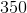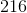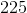Correct answer:Explanation:

Find the pattern of the sequence:

This pattern is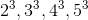so the next number in the sequence would be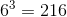### Example Question #1 : Extrapolations

Find the next 2 numbers in this sequence: 33, 46, 72, 111

Possible Answers: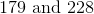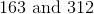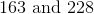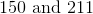Correct answer:Explanation:

Find the pattern in this sequence of numbers:

In this case, the pattern is adding 13n to the previous number where n= how many numbers came before the current number.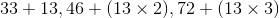so the first number we are looking for would be: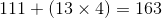the second number we are looking for would be: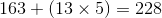### Example Question #3 : Extrapolations

The amount of water inside of a leaky boat is measured periodically after the boat has been in the water in different periods of time and are found to have a linear relationship. The results are given in the following chart:

 Time in water (mins) Amount of water in the boat (gal) 0 0 6 4.8 19 15.2 28 22.4

Using the method of linear extrapolation based on the data from the table, how much water would you expect to be in the boat after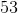minutes?

Possible Answers: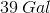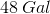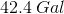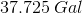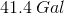Correct answer:Explanation:

To extrapolate the results of the study out to 53 minutes, first we have to determine an equation representing the relationship between time passed and amount of water; we can write our equation in slope-intercept form:Where our y-axis represents amount of water and the x-axis represents time. We can pick 2 points and label them Point 1 and Point 2; looking back at the table:

 Time in water (mins) Amount of water in the boat (gal) 0 0 6 4.8 19 15.2 28 22.4

We label Point 1 as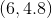and Point 2 as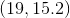; we plug these points into the slope formula as follows: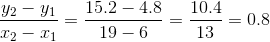So, the slope of our line that describes how much water is in the boat is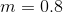; to find ourterm, the y-intercept, we need to pick a point on the graph and plug in our slope to solve for y-intercept. Let's once again choose the point: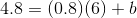Simplify the expression and we find that b=0, so our slope-intercept equation is: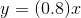Plugging in a value of 53 for, we find that: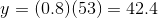So the answer is 42.4 gallons of water in the boat after 53 minutes.

### All Algebra II Resources